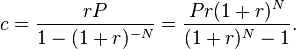# Everyday Calculation

Free calculators and unit converters for general and everyday use.

Calculators » Finance » Loan Tenure

## Loan Term Calculator

Our online tools will provide quick answers to your calculation and conversion needs. On this page, you can calculate time to payoff or loan term for fixed loans including mortgages, student loans and credit cards.

% p.a.

Result window

Download: Use this loan calculator offline with our all-in-one calculator app for Android and iOS.

To calculate loan tenure where payment is made in daily, quarterly or yearly installments, use the present value of annuity calculator. Select "Solve for No. of payments" and enter the loan amount in the present value field.

#### Loan payoff calculation

The loan term or number of monthly payments can be calculated by solving for N in the mortgage formula shown below:Where,
P = principal amount borrowed,
c = periodic payment,
r = monthly interest rate, expressed in decimal (annual interest rate in % divided by 100, and divided by 12)
N = loan term in months

For calculating savings on early loan repayment, click on the calculator linked below.